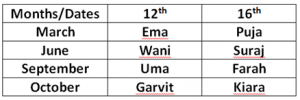Latest Banking jobs   »

# Reasoning Ability Quiz For SIDBI GRADE A 2023- 4th January

Direction (1-3): Study the numbers and letters sequence carefully and answer the questions given below.

X 7 A 0 8 S 9 T 1 4 U 5 0 Q 6 Z 3 2 C 1 8 V 5 B 2 E N 9 6 M 0 3 O 7 F 4 1 I

Q1. Which amongst the following element is 16th to the left of 28th element from the left end?
(a) V
(b) 5
(c) B
(d) 2
(e) None of these

Q2. How many elements are there between ‘Q’ and the one which is second to the right of ‘N’?
(a) Fourteen
(b) Fifteen
(c) Thirteen
(d) Sixteen
(e) None of these

Q3. What is the product of the number which is 4th number from the left end and 7th number from the right end (exclude all the alphabets while counting numbers)?
(a) 48
(b) 18
(c) 81
(d) 54
(e) None of these

Direction (4-7): These questions are based on the following arrangement.

W R – 8 & M P 9 W C N 2 + = X * A 0 % Z Y \$ 1 # 5 K I 4

Q4. How many such symbols are there in the above arrangement which are immediately followed by a letter as well as immediately preceded by a number?
(a) Two
(b) None
(c) Four
(d) Three
(e) None of these

Q5. Which element is exactly between 6th element from right and 11th element from left end?
(a) A
(b) X
(c) %
(d) Z
(e) None of these

Q6. What is the sum of 3rd number from right end and 2nd number from left end?
(a) 12
(b) 8
(c) 15
(d) 10
(e) None of these

Q7. If W R – 8 are written in the reverse order, & M P 9 are written in the reverse order and so on, then in the new arrangement which of following will be exactly between 2 and \$?
(a) X
(b) +
(c) =
(d) *
(e) None of these

Direction (8-10): Read the following alphanumeric series carefully and answer the questions given below.

4 P J 3 % T @ U K 5 V 1 W # Y 2 B 6 & 9 D 8 G © Z

Q8. Which of the following is the sixth to the left of the fifteenth from the left end of the given arrangement?
(a) 2
(b) &
(c) #
(d) K

Q9. How many such numbers are there in the given arrangement, each of which is immediately preceded by a consonant and immediately followed by a symbol?
(a) None
(b) One
(c) Two
(d) Three
(e) More than three

Q10. If the first five elements and the last ten elements are written in the reverse order, which of the following will be the seventh element to the right of the eleventh element from your left?
(a) %
(b) G
(c) Y
(d) 2
(e) B

Direction (11-15): Study the following data carefully and answer the questions accordingly.
Eight people are born on two different dates i.e., 12th and 16th in four different months i.e., March, June, September, and October of the same year. Wani is born in the month of 30 days. Four people are born between Kiara and Wani. Two people are born between Uma and Kiara. Suraj is born either just before or just after Uma but not in the same month. Suraj is born before September. Farah is born immediately before Garvit. Puja is born before Farah but after Ema.

Q11. Who among the following is born in October?
(a) Farah
(b) Puja
(c) Kiara
(d) Ema
(e) Both Kiara and Ema

Q12. How many people are born between Puja and Uma?
(a) Three
(b) Two
(c) One
(d) Four
(e) Five

Q13. Who is born immediately after Puja?
(a) Farah
(b) The one, who is born in June
(c) Garvit
(d) The one, who is born on 16th
(e) Kiara

Q14. Who among the following are born in the same month?
(a) Ema, Wani
(b) Suraj, Farah
(c) Puja, Wani
(d) Farah, Uma
(e) None of these

Q15. Four among the following five are same in a certain way and forms a group, who among the following does not belong to that group?
(a) Puja
(b) Suraj
(c) Farah
(d) Kiara
(e) Garvit

Solutions

S1. Ans. (b)
Sol. Clearly, 5 is 16th to the left of 28th element from the left end.

S2. Ans. (a)
Sol. Clearly, fourteen elements are there between ‘Q’ and the one which is second to the right of ‘N’

S3. Ans. (c)
Sol. 4th number from the left end = 9
6th number from the right end = 9
So, product of these numbers = 9×9=81.

S4. Ans. (a)
Sol. Clearly, two symbols (8&M, 0%Z) are there in the above arrangement.

S5. Ans. (a)
Sol. Clearly, A is exactly between 6th element from right and 11th element from left end.

S6. Ans. (d)
Sol. 3rd number from right end = 1
2nd number from left end = 9
So, sum of these number = 1+9 = 10.

S7 Ans. (b)
Sol. Clearly, + will be exactly between 2 and \$.

S8. Ans. (d)
Sol. Clearly, K is the sixth to the left of the fifteenth from the left end.

S9. Ans. (c)
Sol. Clearly, there are two numbers which is immediately preceded by a consonant and immediately followed by a symbol.

S10. Ans. (b)
Sol. Clearly, G will be the seventh element to the right of the eleventh element from your left.

Solution (11-15):
Sol.S11. Ans. (c)
S12. Ans. (b)
S13. Ans. (b)
S14. Ans. (d)
S15. Ans. (e)## FAQs

#### Congratulations!